## 3.3.5 The preprocessor MA23XX

Preprocessor MA23XX  is used to call module MA2D3E in a simpler manner than in 'batch'. Keyword MA23 of preprocessor APN3XX (module APNOP3, see chapter 2) also results in the utilization of this mesh generator in a conversational form.

MA23XX has the same organization as APNOXX or APN3XX: we start (option Create) by creating a data file, followed by (option Execute) to execute the module using the requests contained in this data file.

The values to be input (parameter, keyword and associated values) by following the scheme given above. We therefore define:

• the geometry with:
• the definition of the lower basis of the "cylinder" (section 0)
• the definition of the different sections (and therefore the layers)
• the transfer of physical attributes of the 2D starting mesh to the 3D items of the resulting mesh

The keywords and data corresponding to the geometric definitions are the following:

• Construction of the basis: Two possibilities exist.

• BASE: The basis is the 2D mesh with side z=value.

• BASE (A): the keyword to activate
• ZINF (R): the side of this section 0

• XYZ23: The basis is the result of a transformation of the 2D mesh via a function (subroutine XYZ23).

• XYZ23 (A): the keyword to activate
• ISEC (I): the index of the upper section to construct (i.e. 0 to ...)
• FONINT (I): option for entering the projection function defining the passing from the 2D mesh to that of the section(s):
• 0: the projection function XYZ23 is given in the form of a subroutine (see chapter 4, module TN2D3D for example);
• 1: a function parser is used; i.e. (see chapter 1, keyword COUR) we must input the data in the following form:
.
'X01(N,X,Y) = function of X and Y and N, the sectional index;'
enclose the expression between ' and terminate is by a ;
.
'Y01(N,X,Y) = function of X, Y and N ;'
.
'Z01(N,X,Y) = function of X, Y and N ;'
.
FIN (A): indicates the end of data for this function

• Construction of sections 1 to n: Several possibilities are offered.

• XYZ23: Total definition of sections p+1 to q from the 2D mesh. Sections p+1 to q result from a transformation of the 2D mesh via function XYZ23. The starting index is p, i.e., the index of the last section which has just been constructed (with the aid of this keyword or via another means)

• XYZ23 (A): the keyword to activate
• ISEC (I): the index of the last section to construct (i.e. q)
• FONINT (I): option for inputing the projection function defining the passing from the 2D mesh to that of sections p+1 to q:
• 0: the projection function XYZ23 is given in the form of a subroutine
• 1: a function parser is used (see above)

• Global definition of the k sections. Two types of figures are possible:

• construction from the data of a section and the definition of k sections by translation, rotation, dilation, etc., of the starting section (index p of the last section having just been constructed);

• TRAN: Translation
• TRAN (A): the keyword to activate
• ISEC (I): the index of the last section to construct
• TX, TY and TZ (R): the translation vector (between 2 sections)

• ROTA: Rotation
• ROTA (A): the keyword to activate
• ISEC (I): the index of the last section to construct (if rotation occurs as a combination with a translation, this information is already known and is not requested for this keyword)
•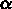(R): the angle of rotation (between 2 sections)
• PX, PY and PZ (R): the coordinates of a point on the rotational axis
• AX, AY and AZ (R): the components of the rotational axis

• DILA: Dilation
• DILA (A): the keyword to activate
• ISEC (I): the index of the last section to construct
•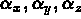(R): the dilation coefficients
• PX, PY and PZ (R): the coordinates of an invariant point

• COMBINE: Combination
• COMBINE (A): the keyword to activate. Put between 2 keywords (and their arguments), this request combines the 2 corresponding transformations. For example TRAN COMBINE ROTA gives a torsion.

• MTRAN: Vertical translation
• MTRAN (A): the keyword to activate
• ISEC (I): the index of the last section to construct
• use array ZINT for indices of the sections to be constructed to stipulate the sides of the sections (see TABL and MANU).

• construction of the k sections by interpolation between an existing section and a section to be defined via function XYZ23 (to obtain this section by transformation of the 2D generic mesh).

• INTER: Interpolation equidistant in z
• INTER (A): the keyword to activate
• ISEC (I): the index of the last section to construct
• FONINT (I): manner in which XYZ23 is input (see above)
• depending on FONINT: XYZ23 data (see above)

• MINTER: Interpolation with the sides given
• MINTER (A): the keyword to activate
• ISEC (I): the index of the last section to construct
• FONINT (I): 0 or 1 and according to this value XYZ23 must be given (as above)
• utilize array ZINT for the indices of the section to construct in order to stipulate the sides of the sections (see TABL and MANU).

• Local definition of section i from section i-1.

• XYZ33: Section i results from the transformation of section i-1 via function XYZ33.
• XYZ33 (A): the keyword to activate
• ISEC (I): the index of the section to construct (i)
• FONINT (I): option for the inputing of the projection function defining the passing from the mesh of section i-1 to that of section i:
1. 0: the projection function, XYZ33, is given in the form of a subroutine
2. 1: a function parser is used (see the example of XYZ23 given above)

In order to create and complete array ZINT, used in certain cases, we have 2 keywords:

• TABL: Declaration of array ZINT.
• TABL (A): the keyword to activate
• LONG (I): its length (number of words)
• MANU: complete this table
• MANU (A): the keyword to activate
• N1 (I): the first index of the value in the table that we entered
• N2 (I): the last index of the value entered
• VAL(.) (R): the values of ZINT for indices N1 to N2.

Furthermore, we can use the following options:

• ANNULE: to delete the last request
• ANNULE-TOUT: to delete all
• ANNULE-COMBINE: to delete request COMBINE

The keywords and data corresponding to the definitions of the physical attributes are the following:

REF: Definition of an operation of type transfer attributes. Where:

• SDSD is the 2D sub-domain number3D sub-domain number
• SDFA is the 2D sub-domain numberreference of the 3D horizontal faces
• ARFA is the 2D edge referencereference of the 3D vertical faces
• ARAR is the 2D edge referencereference of the 3D horizontal edges
• ARAF is the 2D edge referencereference of the 3D horizontal edges and vertical faces
• POAR is the 2D point referencereference of the 3D vertical edges
• POPO is the 2D point referencereference of the 3D points
• POPA is the 2D point referencepoint and vertical edge references
• FASU: assign a number to all the items (faces, edges and points) of the upper face
• FAIN: assign a number to all the items (faces, edges and points) of the lower face (or basis)
• GLOB: direct correspondence of the 2D reference numbers (points and edges) to the 3D items of the vertical faces

In each case, we have the following data (example corresponding to ARFA):

• REF (A): the keyword to activate
• .....
• ARFA (A): the keyword to activate
• ISEC1 (I): the index of the starting section for the assignment
• ISEC2 (I): the index of its arrival section
• N2D (I): the 2D number to consider
• N3D (I): the 3D number associated to the items deduced from the 2D items with number N2D.
• .....
• FIN (A): the keyword to terminate the description of the transfers

Furthermore, we can use the following options:

• ANNULE: to delete the last request
• ANNULE-TOUT: to delete all (for option REF)

The possible options (verification, gluing together, etc.) are requested in the form of keywords:

• VERVOL: verification of the volumes
• EPS: tolerance for this verification
• COLLER: glue together the 2 extreme bases
• NON-VERVOL: cancel VERVOL
• NON-COLLER: cancel COLLER

Lastly, to terminate:

• FIN: End of the construction of the data file

Consult chapter 2 ( MA23) and chapter 5 for examples, or see the examples given below.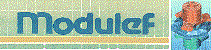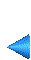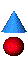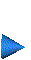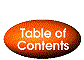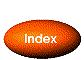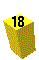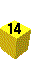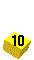Next: 3.3.6 Examples of use Up: 3.3 Extrusion method (cylindrical topology) Prev: 3.3.4 Calling of module MA2D3E Index Contents